# JVM Research and Technology

####Making Ideas Happen

Display Metrology Work - A method to use ∆u'v' as validation for white point from its CCT (Correlated Color Temperature) value

## CCT White-Point Validation

#### Alternate: CCT offset from the Planckian LocusDESCRIPTION: This test procedure is a display metrology method to determine the validation of a CCT white point. It is a combined metric, in that it combines CCT and Δu'v' to determine how valid is a white point. This work is the basis for procedure 5.20 of the ICDM display measurement standard, IDMS1.03.  That standard can be found as a free PDF download on the ICDM web site: http:www.icdm-sid.org.

With the proper Luminance Measurement Device (LMD) we can measure white chromaticity coordinates in terms of u'v' and can determine the Correlated Color Temperature (C.C.T.). CCT has numeric values that define their proximity to the Planckian (black-body) locus. The Planckian locus is a curve that indicates the color characteristic of light that a perfect black body radiator will produce as it is heated.

As a black body radiator is heated, it radiates part of the electromagnetic spectrum that is visible (which is light). The color of the light is a function of the heat of the black body radiator. That color, or CCT, ranges from red for lower values, to blue for higher values. CCT values are in Kelvin (K), as can be seen in Figure 1.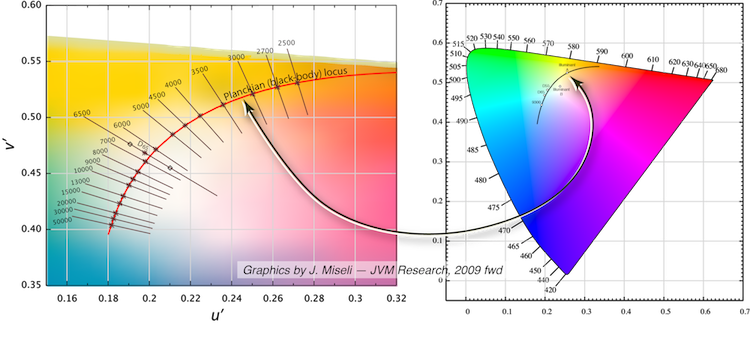Figure 1: 1976 CIE Chromaticity diagram (right side) and a zoomed version (left side) showing the Planckian (black-body) locus with isotemperature lines with lengths of ±2Δu'v' except for the Illuminant D65 line which is lengthened. D65, or a CCT of 6500 K, is a standardized white point used frequently for displays.

CCTs usually lie on the locus.  As shown in Figure 1 left, D65 (6500 K) is actually offset from the locus.  The farther a CCT is from the locus, the less meaningful it is to define a white color.  The diamonds on the isotherm line for illuminant D65 in Figure 1 left give an example.  The upper one tends to be more yellow/green.  The lower one tends to be more red/blue.

It is possible to calculate a CCT number for any color, including saturated colors like Red, Green, Blue, but that gives a value that does not make sense. CCT is meant to indicate white, and must be on or close to the the Planckian locus to be meaningful.

The measurement method presented here calculates a Δu'v' value to determine how far from the black-body curve the CCT value lies. If it is within a specified Δu'v' value then it is a pass and the CCT value can be considered a valid or useful indicator of white. If it is beyond that limit, it fails as a suitable “white” CCT. We do not say what the limit is to be or what numeric results will give a pass or fail. That is up to interested parties, display manufacturers, marketing organizations, etc. We only say how to make the measurement and calculate the result.

In order to perform this process, measure or otherwise determine the white characteristics of a display at the point of interest, typically the center of the screen. Analysis will be performed from these values in terms of the u'v' characteristics. CCT will be calculated or can be provided as input if  the LMD provides a measured value. If CCT can be measured directly or is known from a reliable source, then you can skip directly to step 3 of the process below.

Symbols: u', v'  u'v' (or xy) refer to white chromaticity coordinates. Δu'v' refers to the calculated result of deviation of the u'v' measured results from the u'v' values for a given color temperature (CCT) on the Planckian locus. TW is the variable used for CCT, or the "Temperature" of "White." The variables u'W and v'W are used for the u'v' of the measured white. u'Pv'P are the intermediate calculated points which give an exact point on the Planckian locus closest to the measured u'v' values of u'W and v'W. u'Pv'P thereby become the reference point for how Δu'v' is determine as a distance from the Planckian locus.

APPLICATION: This applies to all displays capable of rendering white. A pair of u'v' chromaticity measurements are needed, but xy coordinates can be used and converted to u'v' as shown for Method B below. xy refers to the CIE 1931 chromaticity diagram. It is not used for modern color measurements, as the CIE 1976 u'v' is the proper coordinate system to use, but it is give herein for convenience only, as some LMD instrumentation measures only xy, and xy can be converted u'v' values per Equation 5 below.

DESCRIPTION: We calculate the Δu'v' distance of a measured white point with chromaticity coordinates (u'Wv'W) from the point on the Planckian (black-body) locus (u'Pv'P) having the same CCT TW . Units: K (kelvin) for CCT, none for Δu'v'. Symbols: (u'Wv'W) for the measured white point, (u'Pv'P) for the point on the Planckian locus having the same CCT.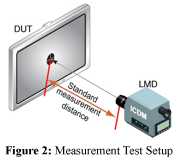SETUP: Figure 2 shows a typical setup for the measurement.  The icons below show a standard setup, where details apply as found in the IDMS (§ 3.2). That is, the icons are used to describe test setup conditions in pictorial form, rather than to have written descriptions of many conditions.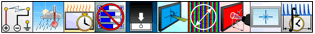PROCEDURE & ANALYSIS: If the white point chromaticity coordinates have already been measured, they need not be measured again in which case proceed to step 2.

1. Measure the chromaticity coordinates (u'W and v'W) of a full-screen white pattern, or one that will not reduce maximum luminance level for a display with luminance loading.

2. Calculate the CCT of TW from the following equation. Here is the full equation in a very small font: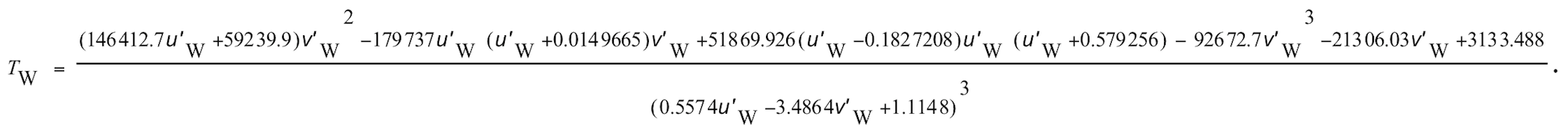(1)

Here is the same equation with the numerator wrapped around for easier viewing. The long numerator is wrapped on two lines.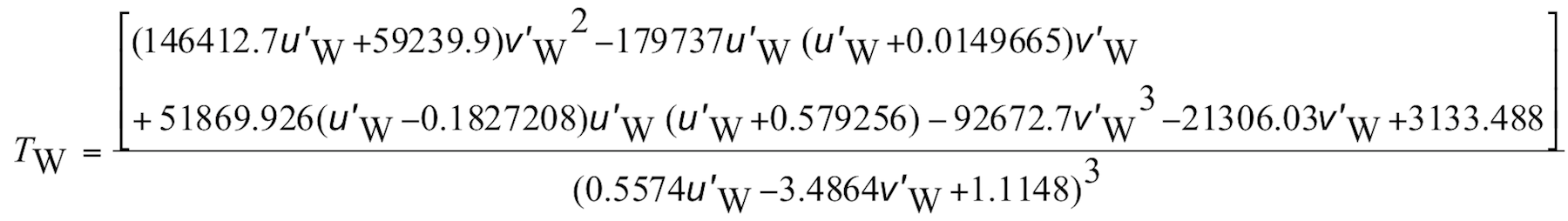(2)

3. From the CCT TW use the following equations to find the chromaticity coordinates (u'Pv'P) for the same CCT on the Planckian locus. Note: Use the u'P and v'P calculations only in the range of 1000 K ≤ TW ≤ 15000 K.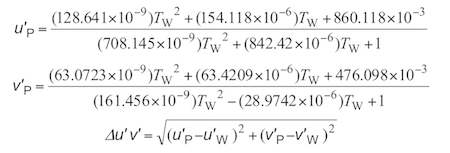(3a)

(3b)

(4)

REPORTING: When this procedure is performed based on measured or other input data, report (u'Wu'W), CCT (TW), and Δu'v'. Optionally specify Δu'v' thresholds, determine if the calculated value is within those limits, and report that as pass or fail.  The table shows sample data taken and the calculated results.

COMMENTS: (1) The Euclidean distance Δu'v' indicates how far off from the Planckian locus the measured (u'wv'w) values are. When we combine the CCT with the Δu'v', we can determine at what limit the CCT is or is not useful or realistic for defining a white point. (2) Equations (2) and (3) are from Krystek, Michael P. (1985). “An algorithm to calculate correlated colour temperature.” Color Research & Application 10 (1): 38–40. doi:10.1002/col.5080100109. Krystek’s equations are for 1960 uv space. In Eqs. (2)-(3), Krystek’s v equation is multiplied by 1.5 to convert it to v'. The u equation is identical for u or u'.

(3) If xW, yW are measured rather than u'wv'w, use the transformation equations to obtain (u'v') as follows (Equation 5):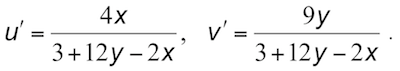(5)

COMMENTS: (2) The Table shows example data only. It is only for reference. Do not use this sample or any values shown to represent expected results of your measurements or display.

 —SAMPLE DATA ONLY— Do not use any values shown to represent expected results of your measurements. Analysis Example Item Measurements Value u'W (u' measured) 0.2056 -- v'W (v'measured) 0.5355 -- Calculations CCT (T) 4090.7 K u'P (u' on locus) 0.2236 -- v'P (v' on locus) 0.4998 -- Δu’v' 0.0399 -- Optional: Specified Δut'vt' threshold 0.xxxx -- Is the calculated Δu'v' within the threshold limit? Δu'v' ≤ Δut'vt' pass / fail --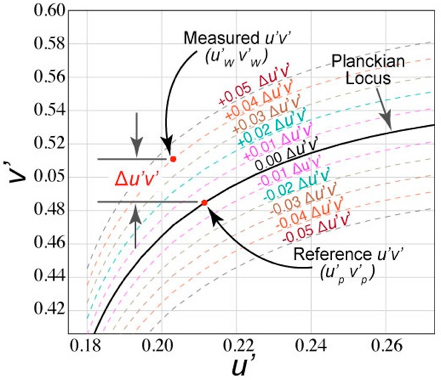Fig. 3. Example showing a measured (u'Wu'W) point and a reference point (u'Pu'P) exactly on the Planckian locus with the Δu'v' showing how far the measured point is from the same CCT point on the Planckian locus.